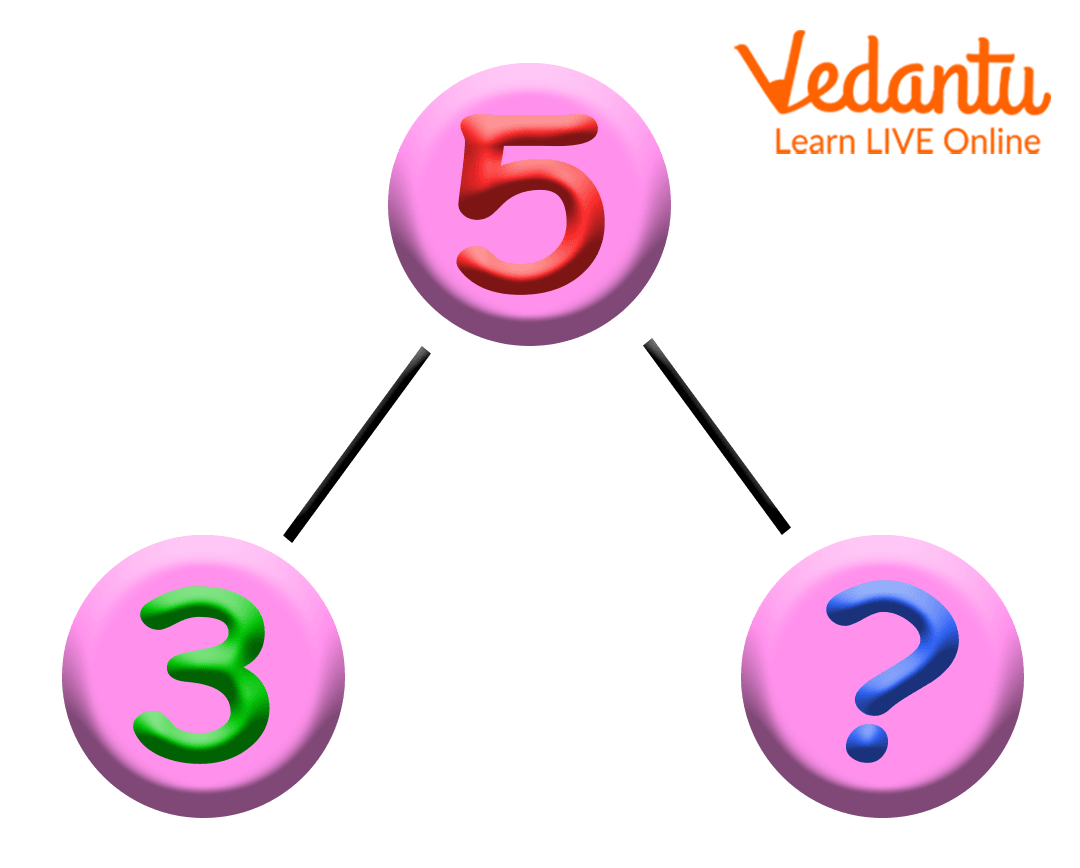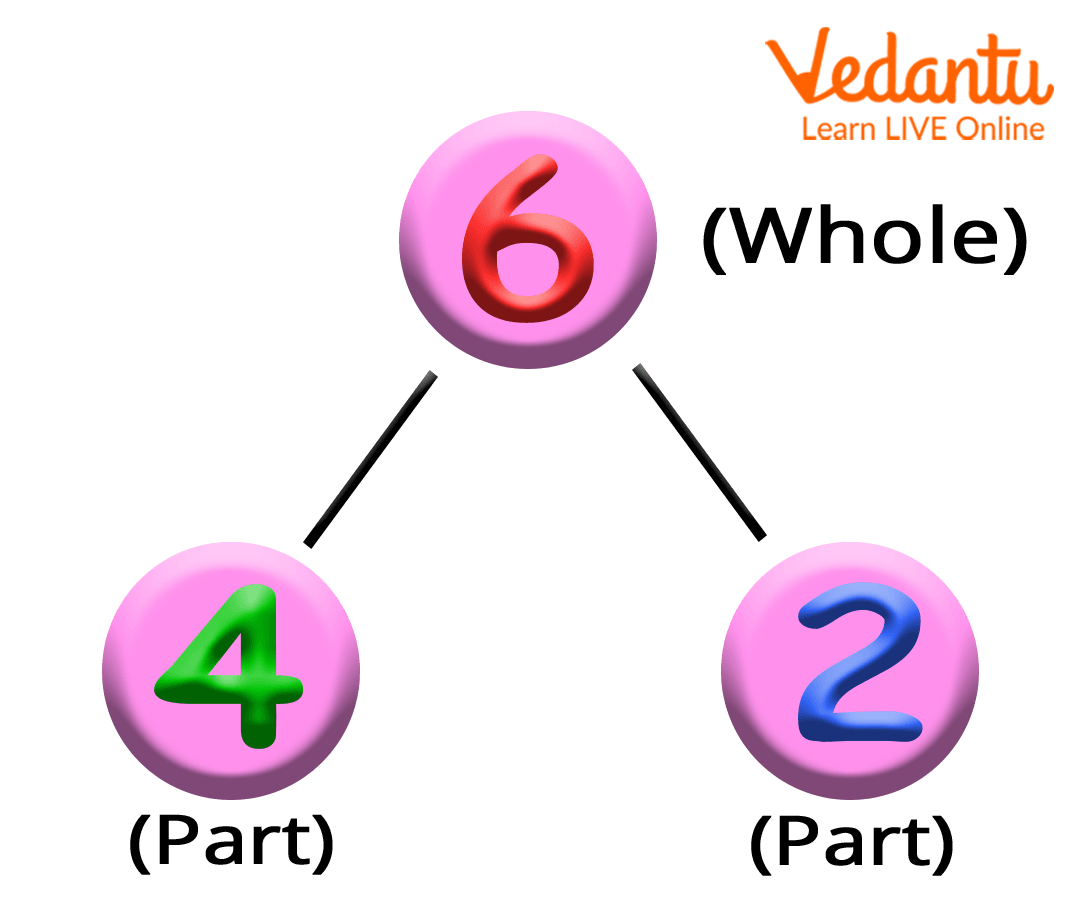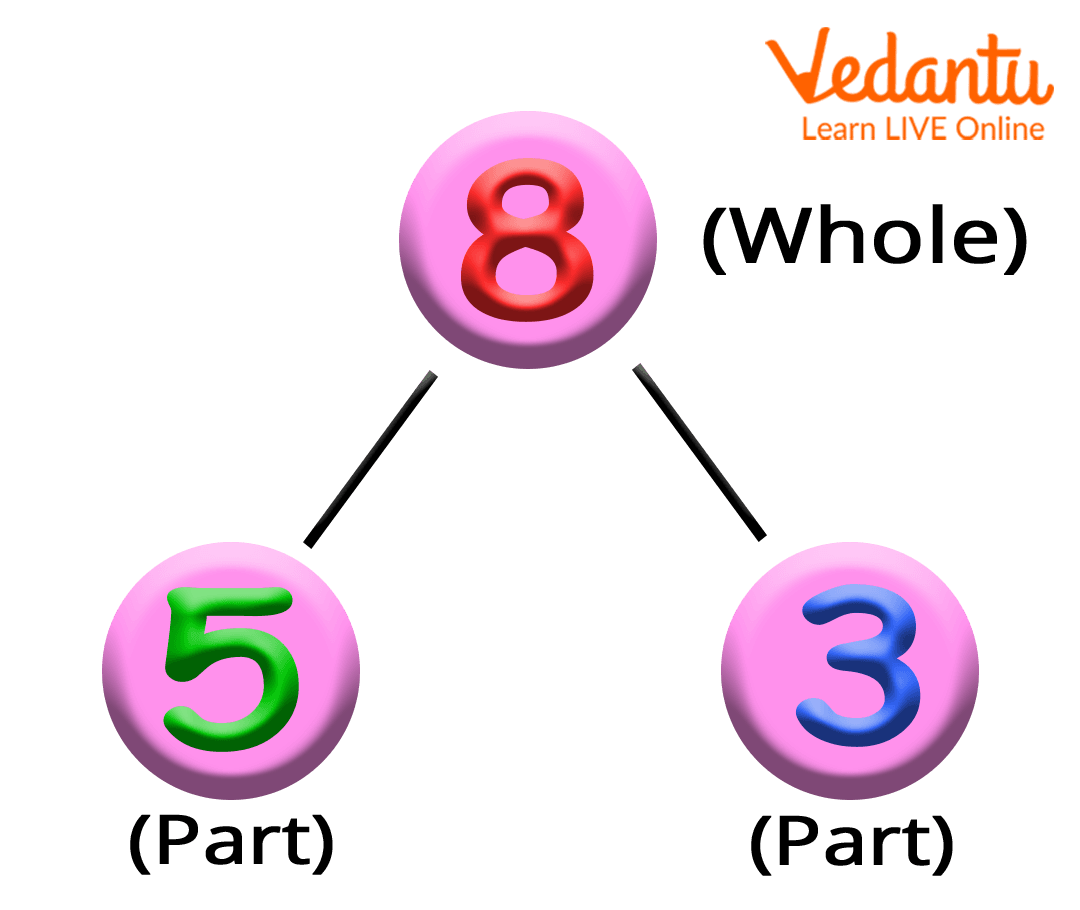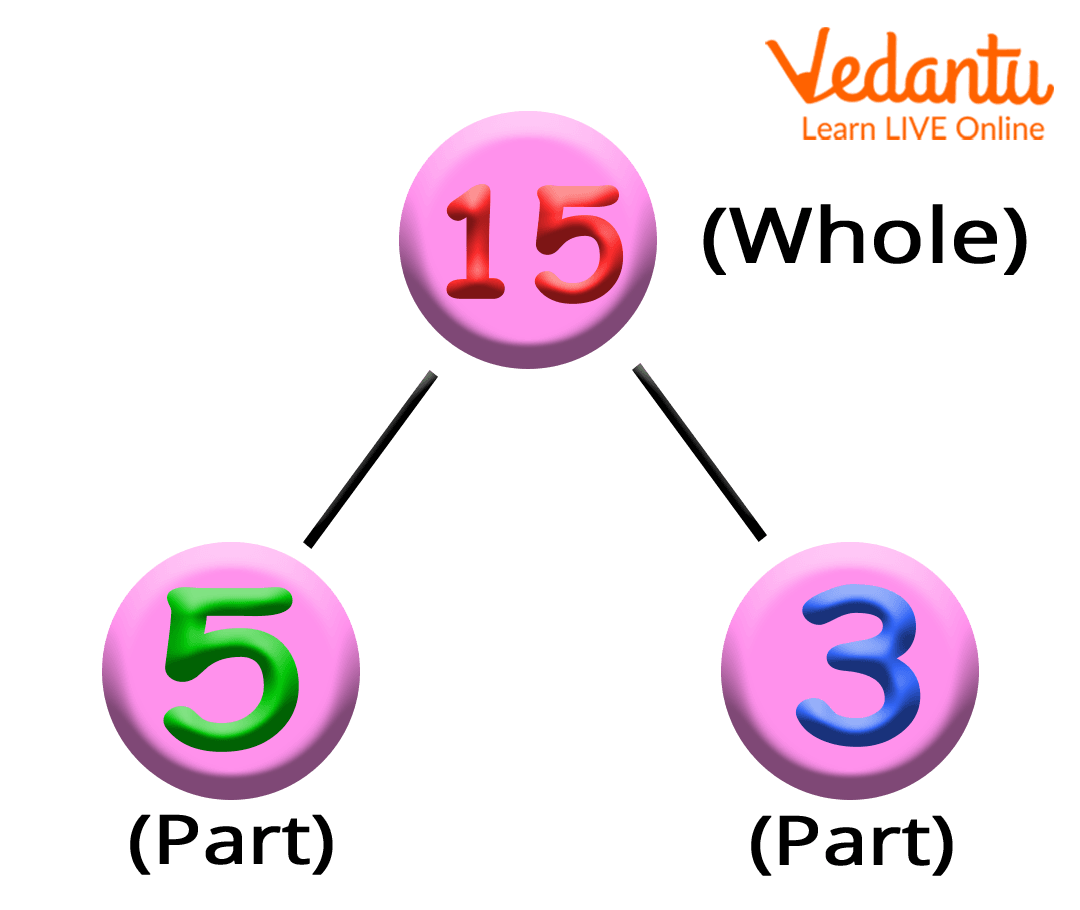Courses
Courses for Kids
Free study material
Free LIVE classes
More

# Number Bond Example: Learn Number Bond with Interactive Examples## Number Bonds Introduction for Kids

Number bonds are pairs of numbers that are added together to form another larger number. This is why they are often referred to as number pairs. For example, a number bond looks like this: 9 + 1 = 10.

Children who are aware of number bonds can easily and speedily solve addition, subtraction, multiplication, and division problems. Also, they can immediately fill any of the three numbers, if any of them are missing, given the other two numbers.Image: Number bond example

## What are Number Bonds?

A number bond is a model that represents the relationship between a number (whole) and its parts. For example, 4 + 2 = 6.Image: A model representing number bond

## Addition and Subtraction Using Numbers Bond

Let us understand how to add and subtract using number bonds or number pairs with the help of an example below:Image: A model representing number bond

Fill the missing numbers using the number bond model given above.

• 8 = 5 + 3

• 8 = 3 + 5

• 8 - 5 = 3

• 8 - 4 = 4

## Multiplication and Division Using Numbers Bond

Let us understand how to multiply and divide using number bonds or number pairs with the help of an example below:Image: A model representing number bond

Fill the missing numbers using the number bond model given above.

• 15 = 3 5

• 15 = 5 3

• 15 3 = 5

• 15 5 = 3

## Number Bond Examples

Following are some of the number bond examples for different numbers:

### Number Bond Examples That Make 5

The number pairs or number bonds that make the number 5 includes:

• 3 and 2 make 5

• 4 and 1 make 5

### Number Bond Examples That Make 10

The number pairs or number bonds that make the number 10 include:

• 0 and 10 make 10

• 1 and 9 make 10

• 2 and 8 make 10

• 3 and 7 make 10

• 4 and 6 make 10

• 5 and 5 make 10

• 6 and 4 make 10

• 7 and 3 make 10

• 8 and 2 make 10

• 9 and 1 make 10

### Number Bonds Examples That Make 20

The number pairs or number bonds that make the number 20 include:

• 0 and 20 make 20

• 1 and 19 make 20

• 2 and 18 make 20

• 3 and 17 make 20

• 4 and 16 make 20

• 5 and 15 make 30

• 6 and 14 make 20

• 7 and 13 make 20

• 8 and 12 make 20

• 9 and 11 make 20

• 10 and 10 make 20

## Activities to Teach Children Number Bonds at Home

Here are the different ways that you use to make your child number bond at home:

1. Give your child 10 different objects such as buttons, sweets, pens, toys, etc., and ask them the questions such as

• What do you add to 5 toys to make them 9?

• You have 10 buttons, you give 4 to your friends. How many buttons will be left will you?

• If you have 10 chocolates and you have to distribute equal chocolates among 5 of your friends, how many chocolates will each friend get?

• If a pack of pens contains 5 pens, how many pens will 2 packs contain?

1. Write a list of 10 numbers and ask your child and check how much time it takes them to look for another number that makes up each pair ( 2 and 8, 5 and 5, and 7 and 3, etc).

2. Print or draw number cards and ask your child to match them into number pairs or number bonds.

## Conclusion

Thus, number bonds are pairs of numbers that are added together to make another larger number. A number bond is the easiest tool for children to learn arithmetic operations such as addition, subtraction, multiplication, and division. Encouraging children to learn number bonds up to 10 or 20 can help them to solve arithmetic calculations accurately and confidently.

Last updated date: 30th Sep 2023
Total views: 138.6k
Views today: 3.38k

## FAQs on Number Bond Example: Learn Number Bond with Interactive Examples

1. How does the number bond in Mathematics work?

Number bonds, also known as number pairs, are pairs of numbers that are added together to obtain another number. Number bonds provide the relationship between two numbers through a written model that shows how numbers are related.

2. Why is it important for kids to learn number bonds?

Number bonds are important when it comes to solving complex calculations involving addition and subtraction. For example, knowing the fact that 6 and 4 make 10,  will easily help you to calculate 60 + 40 because you know that 6 and 4 make 10 but adding one zero at the end will give you the answer 100.

You can easily get this without much calculation if you know that 6 + 4 is 10 and also are aware of the place value concept. Hence, children having perfect knowledge of number bonds can easily and precisely perform arithmetic calculations.